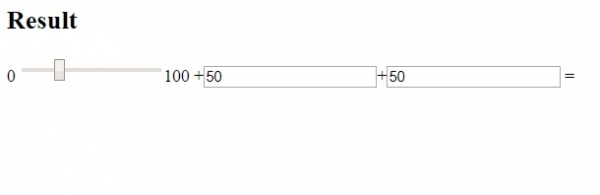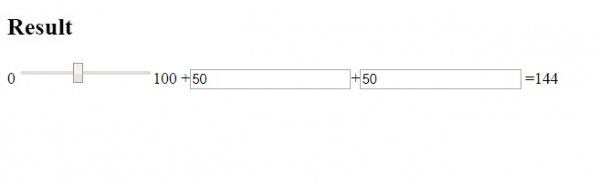# HTML <output> for Attribute

HTMLWeb DevelopmentFront End Technology

The for attribute of the <output> element sets the relationship between the result of the calculation and the elements used in the calculation.

Following is the syntax:

<output for="id">

Above, id is the element id, which sets a separate list of one or more elements with a space. These elements specify the relationship between the result of the calculation and the elements used in the calculation.

Let us now see an example to implement the for attribute of the <output> element:

## Example

Live Demo

<!DOCTYPE html>
<html>
<body>
<h2>Result</h2>
<form oninput="n.value=parseInt(p.value)+parseInt(q.value)+parseInt(r.value)">0
<input type="range" id="p" value="25">100+<input type="number"
id="q" value="50">+<input type="number" id="r" value="50">
=<output name="n" for="p q r"></output>
</form>
</body>
</html>

## OutputNow, increase the slider and the result would get displayed: